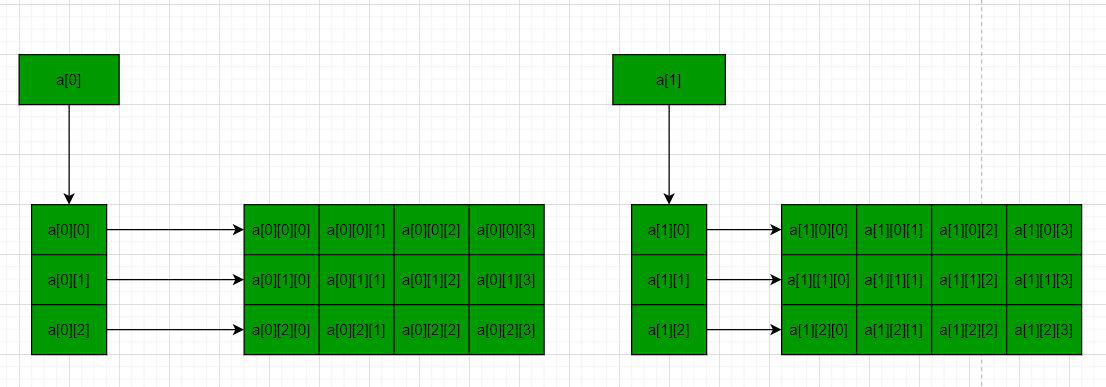# How to dynamically allocate a 3D array in C++

• Difficulty Level : Expert
• Last Updated : 24 Mar, 2021

Prerequisite: Array Basics
In C/C++, multidimensional arrays in simple words as an array of arrays. Data in multidimensional arrays are stored in tabular form (in row major order). Below is the general form of declaring N-dimensional arrays:

Syntax of a Multidimensional Array:

data_type  array_name[size1][size2]….[sizeN];

data_type: Type of data to be stored in the array.
Here data_type is valid C/C++ data type
array_name: Name of the array
size1, size2, …, sizeN: Sizes of the dimensions

3-D arrays are an array of Double dimensional arrays:

Syntax of a 3D array:

data_type array_name[x][y][z];
data_type: Type of data to be stored. Valid C/C++ data type.

For more details on multidimensional and, 3D arrays, please refer to the Multidimensional Arrays in C++ article.

Problem: Given a 3D array, the task is to dynamically allocate memory for a 3D array using new in C++.

Solution: In the following methods, the approach used is to make two 2-D arrays and each 2-D array is having 3 rows and 4 columns with the following values.

```1 2 3 4
5 6 7 8
9 10 11 12

13 14 15 16
17 18 19 20
21 22 23 24```

X = No of 2D arrays.
Y = No of rows of each 2D array.
Z = No of columns of each 2D array.

Method 1: using single pointer – In this method, a memory block of size x*y*z is allocated and then the memory blocks are accessed using pointer arithmetic. Below is the program for the same:

## C++

 `// C++ program to dynamically allocate` `// the memory for 3D array in C++` `// using new operator` `#include ` `using` `namespace` `std;`   `// Driver Code` `int` `main()` `{` `    ``// Dimensions of the 3D array` `    ``int` `x = 2, y = 3, z = 4;` `    ``int` `count = 0;`   `    ``// Allocate memory blocks` `    ``// of size x*y*z` `    ``int``* a = ``new` `int``[x * y * z];`   `    ``// Traverse the 3D array` `    ``for` `(``int` `i = 0; i < x; i++) {` `        ``for` `(``int` `j = 0; j < y; j++) {` `            ``for` `(``int` `k = 0; k < z; k++) {`   `                ``// Assign values to the` `                ``// memory blocks created` `                ``*(a + i * y * z + j * z + k) = ++count;` `            ``}` `        ``}` `    ``}`   `    ``// Traverse the 3D array again` `    ``for` `(``int` `i = 0; i < x; i++) {` `        ``for` `(``int` `j = 0; j < y; j++) {` `            ``for` `(``int` `k = 0; k < z; k++) {`   `                ``// Print values of the` `                ``// memory blocks created` `                ``cout << *(a + i * y * z + j * z + k) << ``" "``;` `            ``}` `            ``cout << endl;` `        ``}` `        ``cout << endl;` `    ``}`   `    ``// Deallocate memory` `    ``delete``[] a;`   `    ``return` `0;` `}`

Output:

```1 2 3 4
5 6 7 8
9 10 11 12

13 14 15 16
17 18 19 20
21 22 23 24```

Method 2: using triple pointer – Below is the diagram to illustrate the concept:Below is the program for the same:

## C++

 `// C++ program to dynamically allocate` `// the memory for 3D array in C++` `// using new operator` `#include ` `using` `namespace` `std;`   `// Driver Code` `int` `main()` `{` `    `  `    ``// Dimensions of the 3D array` `    ``int` `x = 2, y = 3, z = 4;` `    ``int` `count = 0;`   `    ``// Allocate memory blocks of size` `    ``// x i.e., no of 2D Arrays` `    ``int``*** a = ``new` `int``**[x];`   `    ``for` `(``int` `i = 0; i < x; i++) {`   `        ``// Allocate memory blocks for` `        ``// rows of each 2D array` `        ``a[i] = ``new` `int``*[y];`   `        ``for` `(``int` `j = 0; j < y; j++) {`   `            ``// Allocate memory blocks for` `            ``// columns of each 2D array` `            ``a[i][j] = ``new` `int``[z];` `        ``}` `    ``}`   `    ``for` `(``int` `i = 0; i < x; i++) {` `        ``for` `(``int` `j = 0; j < y; j++) {` `            ``for` `(``int` `k = 0; k < z; k++) {`   `                ``// Assign values to the` `                ``// memory blocks created` `                ``a[i][j][k] = ++count;` `            ``}` `        ``}` `    ``}`   `    ``for` `(``int` `i = 0; i < x; i++) {` `        ``for` `(``int` `j = 0; j < y; j++) {` `            ``for` `(``int` `k = 0; k < z; k++) {`   `                ``// Print values of the` `                ``// memory blocks created` `                ``cout << a[i][j][k] << ``" "``;` `            ``}` `            ``cout << endl;` `        ``}` `        ``cout << endl;` `    ``}`   `    ``// Deallocate memory` `    ``for` `(``int` `i = 0; i < x; i++) {` `        ``for` `(``int` `j = 0; j < y; j++) {` `            ``delete``[] a[i][j];` `        ``}` `        ``delete``[] a[i];` `    ``}` `    ``delete``[] a;`   `    ``return` `0;` `}`

Output:

```1 2 3 4
5 6 7 8
9 10 11 12

13 14 15 16
17 18 19 20
21 22 23 24```

My Personal Notes arrow_drop_up
Recommended Articles
Page :